Published

# Industrial and Home Security System

It system fully based on the security purpose which is use in INDUSTRY, FURNACE, HOME, MUSEUM and CAR security.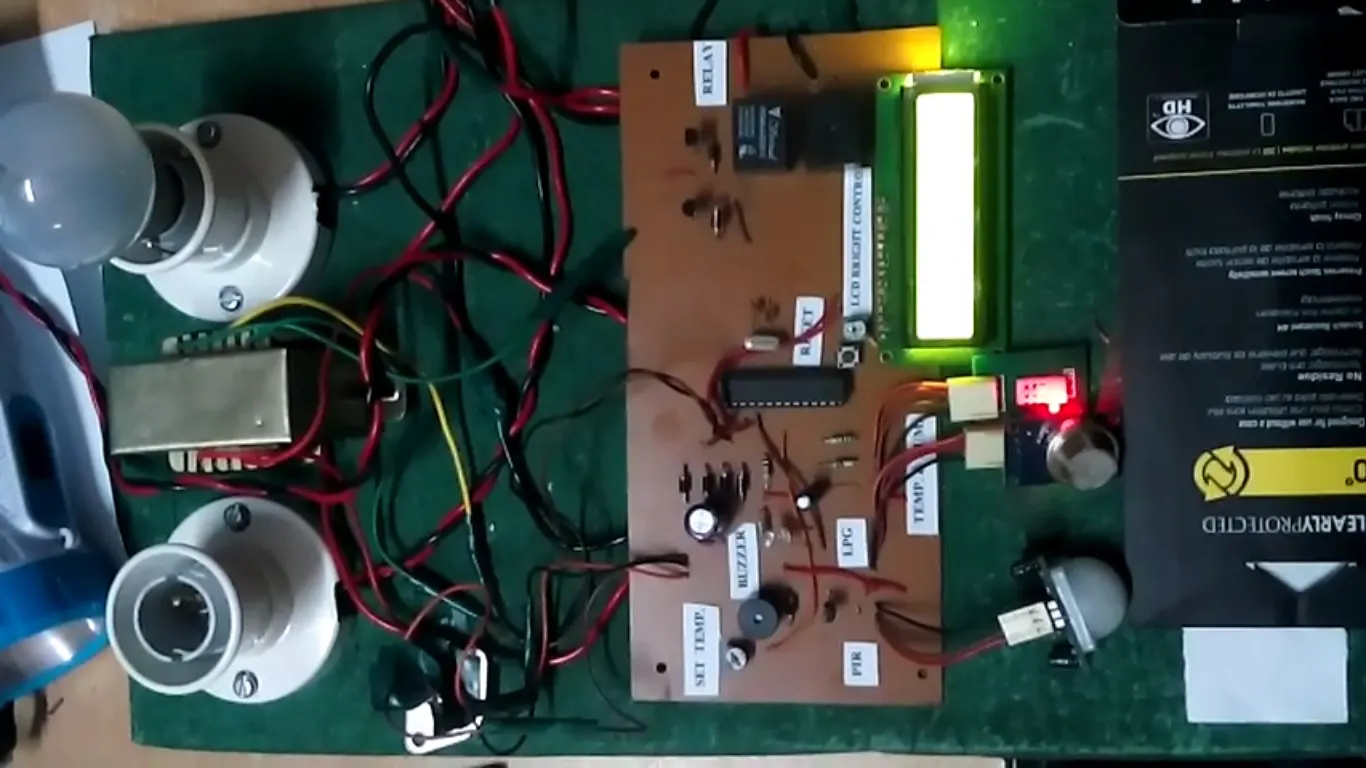## Things used in this project

### Hardware components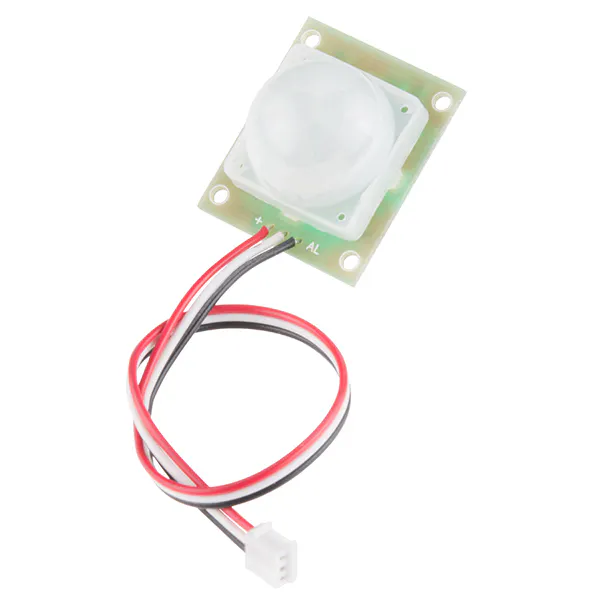PIR Motion Sensor (generic)
×1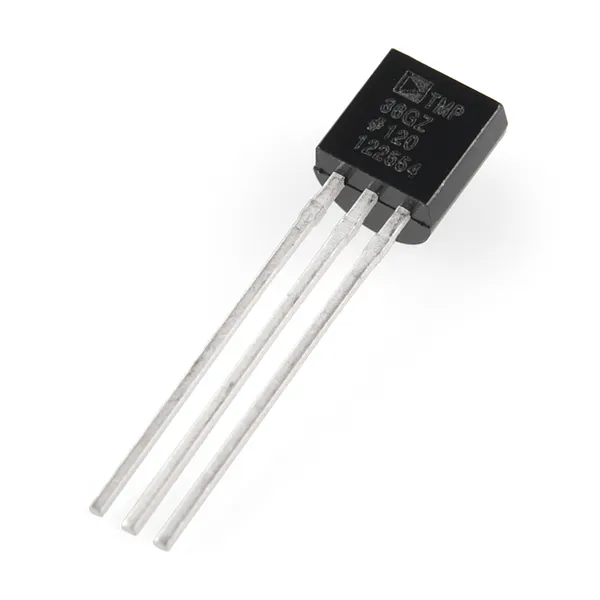Temperature Sensor
×1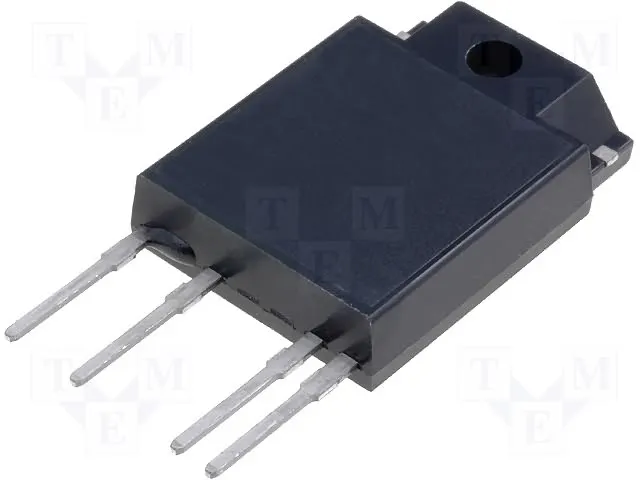Solid State Relay
×1Resistor 10k ohm
×1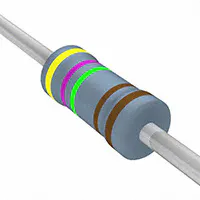Resistor 4.75k ohm
×1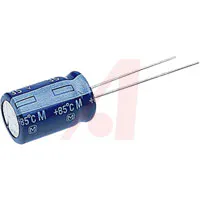Capacitor 1000 µF
×1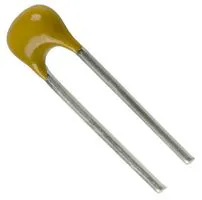Capacitor 22 pF
×1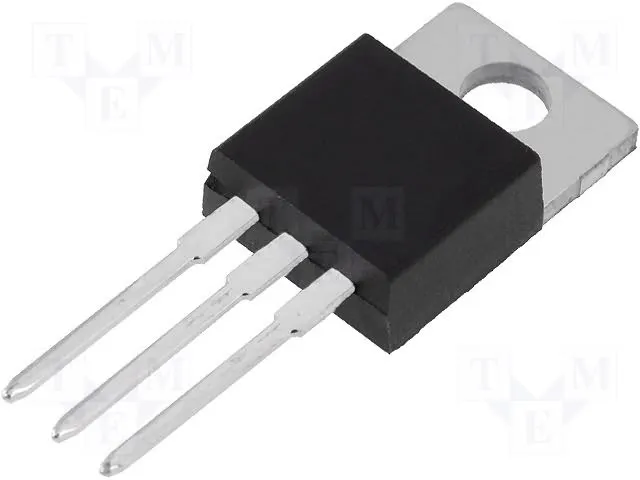Linear Regulator (7805)
×1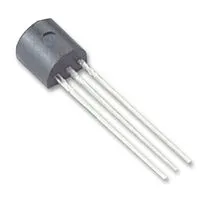General Purpose Transistor NPN
×1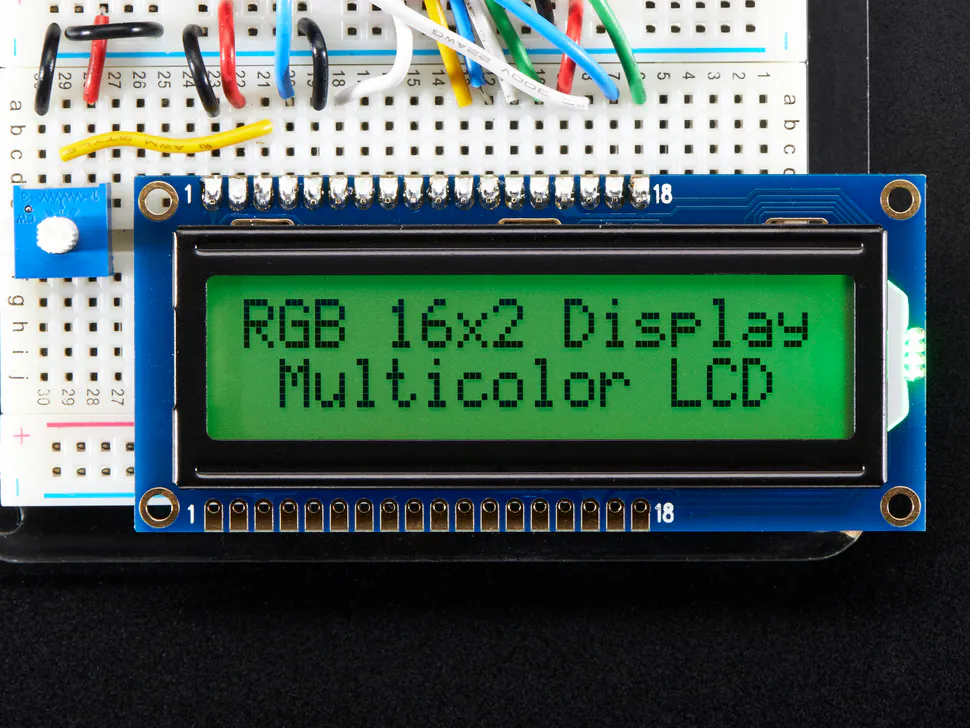Adafruit RGB Backlight LCD - 16x2
×1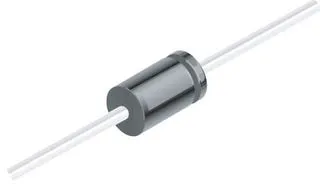1N4007 – High Voltage, High Current Rated Diode
×1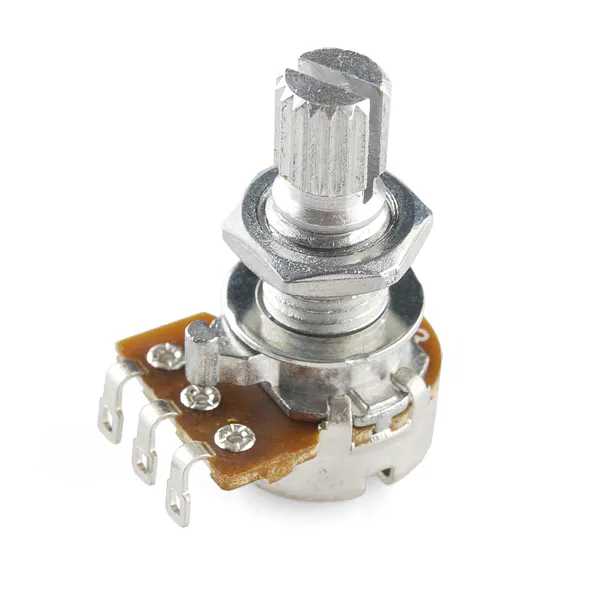Rotary potentiometer (generic)
×1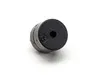Buzzer
×1

### Hand tools and fabrication machinesSoldering iron (generic)

## Schematics

### Circuit Diagram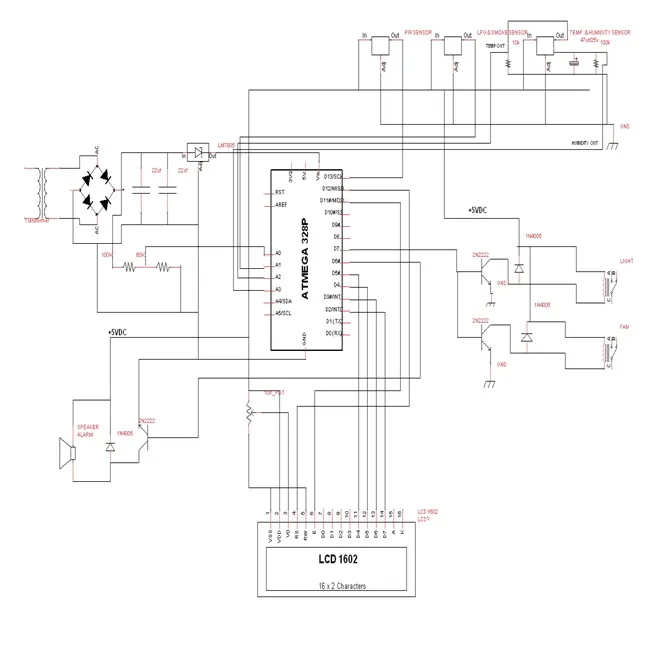### PCB Layout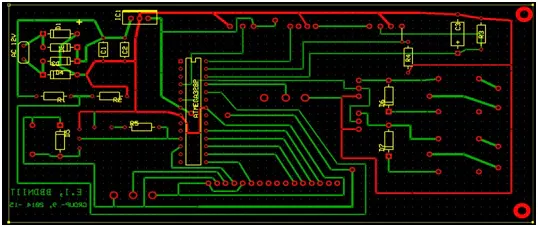### Block Diagram of Project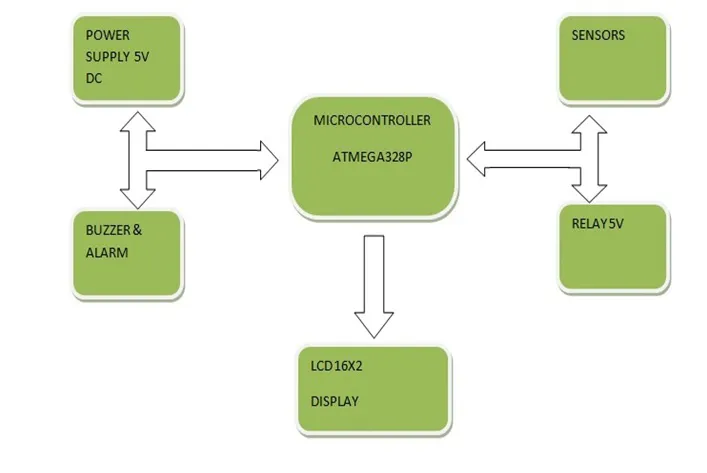## Code

### Code of Project

Arduino
``` // LPG PIN
//LPG AND SMOKE PIN DETECT ALARM

void setup()
{

Serial.begin(9600);
pinMode(13,OUTPUT);
pinMode (12,OUTPUT); //PIR OUTPUT SINGNAL
pinMode (3, INPUT);  // PIR INPUT PIN
int tempsense = 0;
int rhsense = 0;
int temp = 0;
int rh = 0;

}

void loop()
{
{
goto LPG_ND_SMOKE;

goto TEMP_AND_HUMIDITY;

goto PIR;

goto HighVoltage;

}

LPG_ND_SMOKE:
{
int ledPin = 7;
int sensorValue = 0;

if (sensorValue >=550)

{
digitalWrite(ledPin, HIGH);
}
else if (sensorValue>=400)

{
digitalWrite(ledPin, HIGH);
}
else

digitalWrite(ledPin, LOW);

Serial.print("sensor = " );
Serial.println(sensorValue);

delay(10);
}

PIR:
{
int val=0;
digitalWrite(13,val);

if (val == 1)
{
digitalWrite(13,LOW);
}

else
{
Serial.print("LOW PIR" );
}

}

TEMP_AND_HUMIDITY:
{
int tempsense = 0;
int rhsense = 0;
int temp = 0;
int rh = 0;

temp = (tempsense/50);
rh = ((30.855*(rhsense/204.6))-11.504);

Serial.print("Temperature: ");
Serial.print(temp);
Serial.println(" C ");

delay(100);
Serial.print("Humidity: ");
Serial.print(rh);
Serial.println("% ");

delay(1000);
}

HighVoltage:
{
int  max_volt = 255;
int present_main_volt;
float volt;
volt=(5/ max_volt* present_main_volt);

if (volt ==00)
if(volt ==3.177)
if(volt ==3.50)
if(volt==4.00)
if(volt== 4.50)
goto OFF;

}

OFF:
{
digitalWrite(7,HIGH);
delay(1000);
digitalWrite(7,LOW);
}

}
```

## Credits

### rahulwa27

1 project • 1 follower